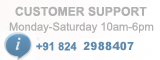# PIC Development Board

/*
* Project name:RELAY INTERFACE
PIC Development Board
(c) Researchdesignlab.com
* Test configuration:
MCU: PIC16F877A
Dev.Board: PIC
Oscillator: 20.0 MHz
Software: MPLAB IDE v8.92(HI-TECH_C)
*/
#define _XTAL_FREQ 20000000 //crystal frequency of 20MHZ
#include "uart.h" //header file
#include "string.h" //header file
#define relay1 RB1
#define relay2 RB2
#define relay3 RB3
#define relay4 RB4

char UART_Init(const long int baudrate)
{
unsigned int x;
x = (_XTAL_FREQ - baudrate*64)/(baudrate*64);
if(x>255)
{
x = (_XTAL_FREQ - baudrate*16)/(baudrate*16);
BRGH = 1; //High Baud Rate Select bit set to high
}
if(x<256)
{
SPBRG = x; //Writing SPBRG register
SYNC = 0; //Selecting Asynchronous Mode
SPEN = 1; //enables serial port
TRISC7 = 1;
TRISC6 = 1;
CREN = 1; //enables continuous reception
TXEN = 1; //enables continuous transmission
return 1;
}
return 0;
}

char UART_TX_Empty()
{
return TRMT; //Returns Transmit Shift Status bit
}

{
return RCIF; //Flag bit
}

char UART_Read() //this function is used to read a byte
{
while(!RCIF); //Waits for Reception to complete
return RCREG; //Returns the 8 bit data
}
void UART_Read_Text(char *Output, unsigned int length)//this function is used to read a text
{
int i;
for(int i=0;i<length;i++)
}

void UART_Write(char data) //this function is used to write a byte
{
while(!TRMT);
TXREG = data; //transmit register
}

void UART_Write_Text(char *text) //this function is used to write a string
{
int i;
for(i=0;text[i]!='\0';i++)
UART_Write(text[i]);
}

void main()
{
unsigned char ReceivChar;
TRISB=0X00; //make register as the output
PORTB=0X00; //make the PORTB as the output port

UART_Init(9600); //inititalise the UART

DelayMs(1000); //provide delay of 1s

while(1)
{
if(UART_Data_Ready()) //check if the data is ready
{
ReceivChar = UART_Read(); //store the data in a variable
UART_Write(ReceivChar); //display on hyperterminal
__delay_ms(1000); //provide delay of 1s

if(ReceivChar=='1') //check if the received char is 1if 1
{
ReceivChar = UART_Read(); //store the data in a variable
UART_Write(ReceivChar); //display on hyperterminal

if(ReceivChar=='N') //if received character is N
relay1=1; //turn ON the 1st relay
else if(ReceivChar=='F') //if received character is F
relay1=0; //turn OFF the 1st relay
}

else if(ReceivChar=='2') //check if the received char is 2if 2

{
ReceivChar = UART_Read(); //store the data in a variable
UART_Write(ReceivChar); //display on hyperterminal
if(ReceivChar=='N') //if received character is N
relay2=1; //turn ON the 2nd relay
else if(ReceivChar=='F') //if received character is F
relay2=0; //turn OFF the 2nd relay
}

else if(ReceivChar=='3') //check if the received char is 3if 3
{
ReceivChar = UART_Read();//store the data in a variable
UART_Write(ReceivChar); //display on hyperterminal
if(ReceivChar=='N') //if received character is N
relay3=1; //turn ON the 3rd relay
else if(ReceivChar=='F') //if received character is N
relay3=0; //turn OFF the 3rd relay
}
else if(ReceivChar=='4') //check if the received char is 4if 4
{
ReceivChar = UART_Read();//store the data in a variable
UART_Write(ReceivChar); //display on hyperterminal
if(ReceivChar=='N') //if received character is N
relay4=1; //turn ON the 4th relay
else if(ReceivChar=='F') //if received character is N
relay4=0; //turn OFF the 4th relay
}